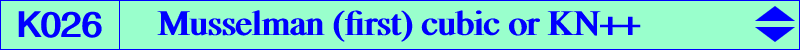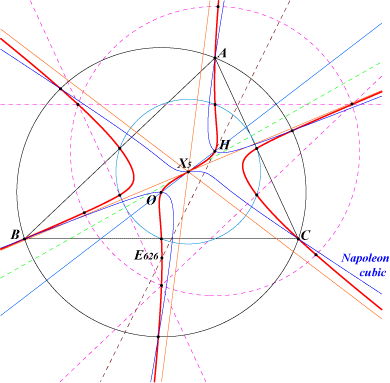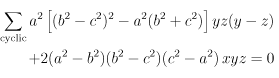X(3), X(4), X(5), X(5403), X(5404), X(8798) midpoints of BC, CA, AB and AH, BH, CH reflections of A, B, C in X(5) i.e. centers of the Johnson circles points on the circumcircle and on the Napoleon cubic. infinite points of the McCay cubic points Na, Nb, Nc mentioned in Central Cubics X3-OAP points, see Table 53We meet this K60++ cubic in a paper by Musselman (see bibliography) and in a totally different context in Special isocubics §6.5.3. K026 is a central equilateral cubic with center X(5), the nine point center, with inflexional tangent X(5)X(51) i.e. the Euler line of the orthic triangle. Its three asymptotes are perpendicular to the sidelines of the Morley triangle and parallel to those of the McCay cubic. It is the homothetic of K080 = KO++ under h(H,1/2) and the isogonal transform of K361. K026 is also psK(X51, X2, X3) in Pseudo-Pivotal Cubics and Poristic Triangles. See also Table 50 and Table 76. K026 is spK(X3, X140) as in CL055. See also Table 54 where K026 is mentioned in one line and three columns showing that it belongs to four pencils of cubics generated by : • K006 and K187, line Q = X140, • K002 and K009, column P = aaQ, • K003 and the union of the line at infinity with the Jerabek hyperbola, column P = X3, • K006 and K080, column P = S. Locus properties : Denote by A1, B1, C1 the reflections of P in A, B, C and by A2, B2, C2 the reflections of P in the sidelines BC, CA, AB. The three circles AB1C1, BC1A1, CA1B1 have a common point D on the circumcircle. The three circles AB2C2, BC2A2, CA2B2 have also a common point N on the circumcircle. These points D, N coincide if and only if P lies on the cubic K026 (Musselman, Some loci connected with a triangle. Monthly, p.354-361, June-July 1940). On the other hand, they are antipodes if and only if P lies on the cubic K226 we call Musselman (fourth) cubic. K026 is also the locus of centers of central pKs whose asymptotes are perpendicular to the sidelines of the Morley triangle or, equivalently, parallel to those of the McCay cubic K003. K026 is the locus of the centers of central focal circum-cubics passing through H. See K530 (center H) and K465 (center X5) for instance. See the related quartics Q019 and Q089. K026 is the locus of the centers of central circum-stelloids passing through H and/or having asymptotes parallel to those of the McCay cubic K003. It is the case of K026 itself (center X5), K080 (center X3) and K525 (center X4). If "parallel" is replaced with "perpendicular", the locus is K607. See Table 33 where K026 is the cubic Lk when k = 1/2. K026 is the Laplacian of the quintic Q038 i.e. the locus of points whose polar conic in the quintic is a rectangular hyperbola.